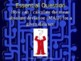6th Grade Statistics & Data 6 - Mean Absolute Deviation Powerpoint LessonSubject
Common Core State Standards
Product Rating
4.0
7 Ratings
File Type

Presentation (Powerpoint) File

Be sure that you have an application to open this file type before downloading and/or purchasing.

3 MB|45 pages
Share
Also included in:
1. Sixth Grade Statistics and Data Bundle is composed of 6 different lessons comprising 361 slides on the following: Statistics and Data 1 - Statistical Questions and Types of Data, Statistics and Data 2 - Mean, Median, Mode & Range, Statistics and Data 3 - Dot Plot and Data Distribution, Statisti
\$19.50
\$15.60
Save \$3.90
2. Sixth Grade Powerpoint Math Bundle is composed of 55 different high quality math powerpoint lessons comprising 2,736 slides. There are 13 strategies of effective teaching based on research in these powerpoint lessons. . For further information on each lesson click on them individually. This is a
\$178.75
\$143.00
Save \$35.75
Product Description

Sixth Grade Statistics 6 - Mean Absolute Deviation teaches students the steps to take to find the Mean Absolute Deviation of a set of data. The skills necessary to do this task are reviewed: how to find the mean, how to find absolute values of numbers, and how to add and subtract integers. There are 13 strategies of effective teaching based on research. For best results, students should have white boards and dry erase markers. Included in this powerpoint lesson are the following:

• title slide
• focus slide
• objectives
• Students will be able to calculate the mean absolute deviation (MAD) for a given data set.
• Students will be able to interpret the MAD as the average distance of data values from the mean.
• essential question
• How can I calculate the mean absolute deviation (MAD) for a given data set.
• vocabulary
• concept development
• step-by-step demonstrations on how to calculate the mean absolute deviation and how to use that information to solve problems
• problem solving with word problem
• reward slide

Be sure to follow my store to be alerted of new products. The first 48 hours is always 50% off. CLICK HERE

Don't forget to leave feedback on this lesson to earn points for purchasing other TpT products.

You may be interested in the following related powerpoint lesson:

Link - 6th Grade Statistics 1 - Introduction, Statistical Questions, and Types of Data

Link - 6th Grade Statistics 2 - Mean, Median, Mode and Range

Link - 6th Grade Statistics 3 - Dot Plot and Data Distribution

Link - 6th Grade Statistics 4 - Histogram

Link - 6th Grade Statistics 5 - Box and Whisker Plot

Total Pages
45 pages
N/A
Teaching Duration
1 hour
Report this Resource to TpT
Reported resources will be reviewed by our team. Report this resource to let us know if this resource violates TpT’s content guidelines.